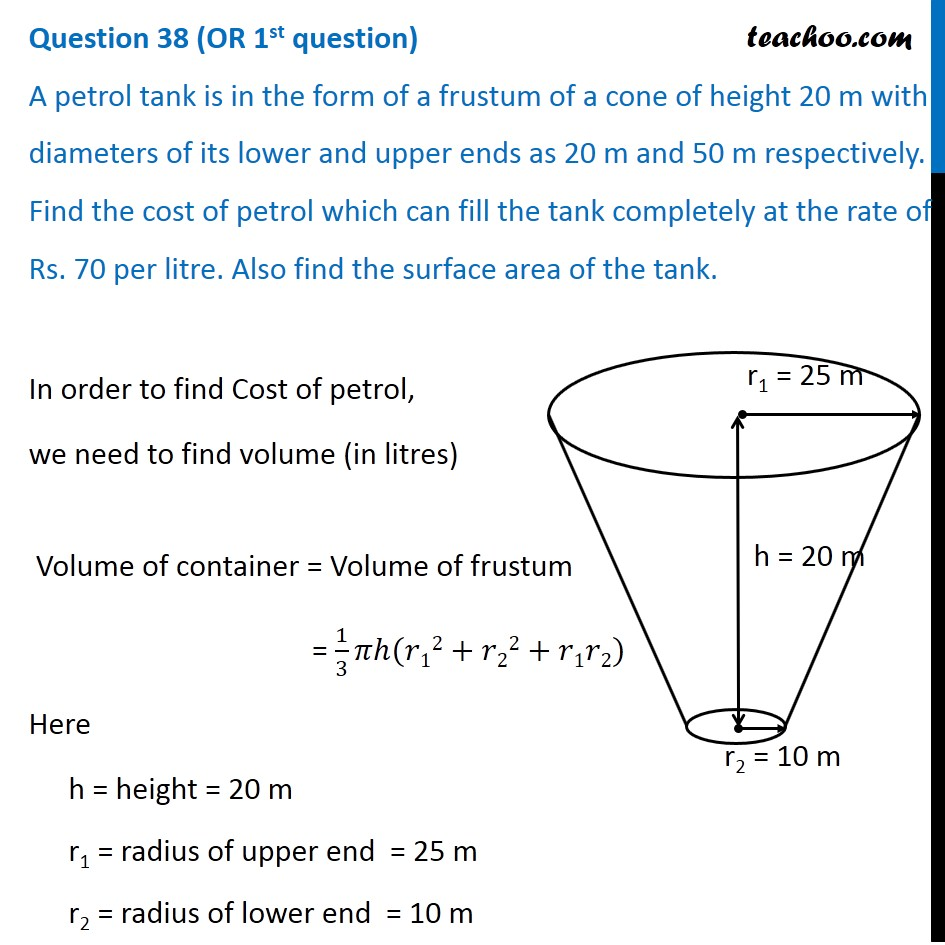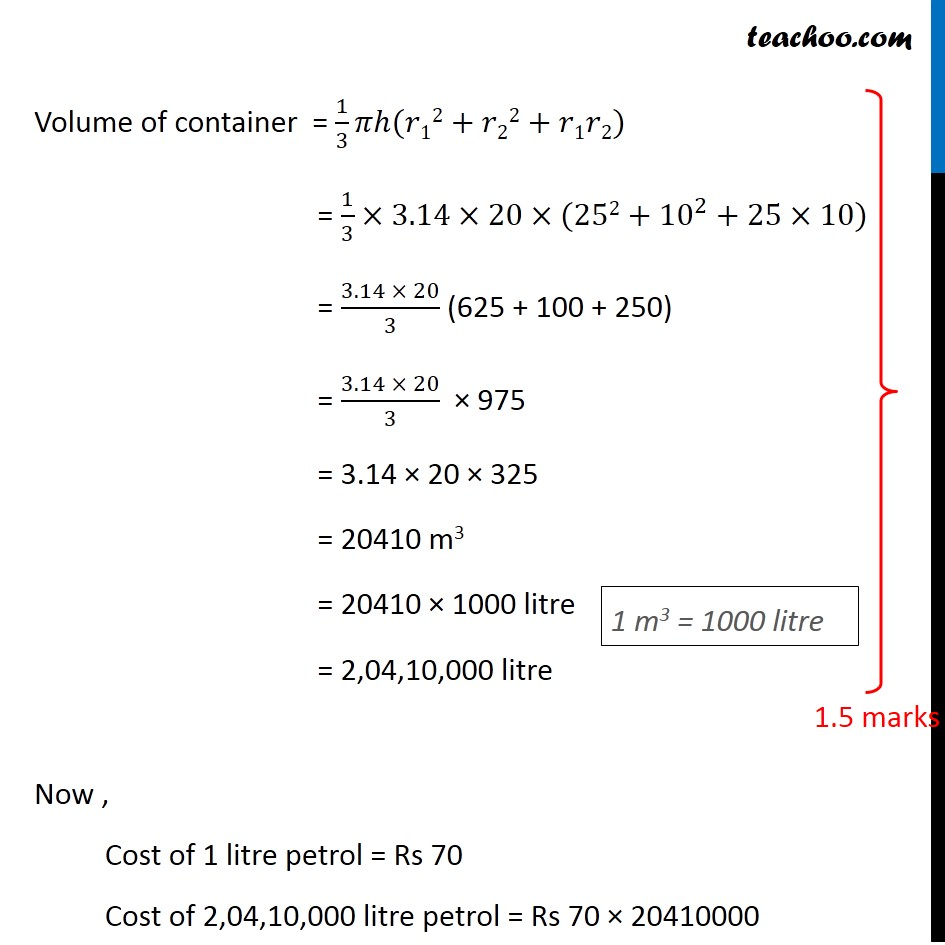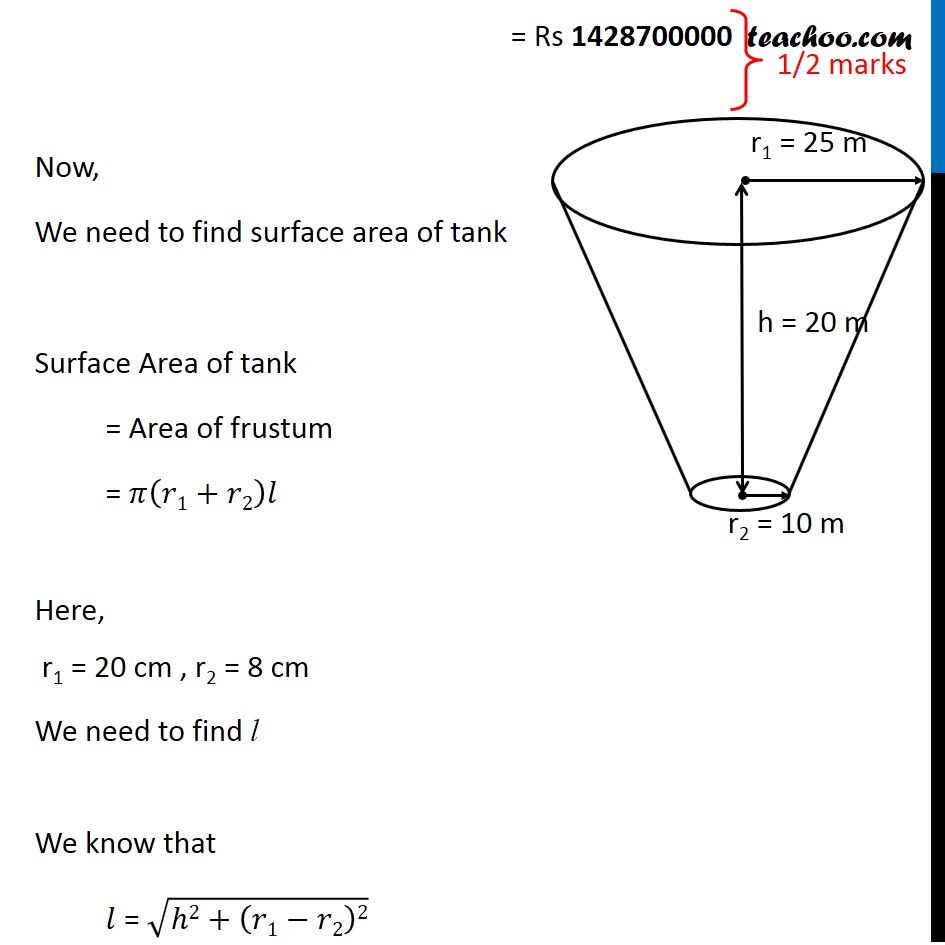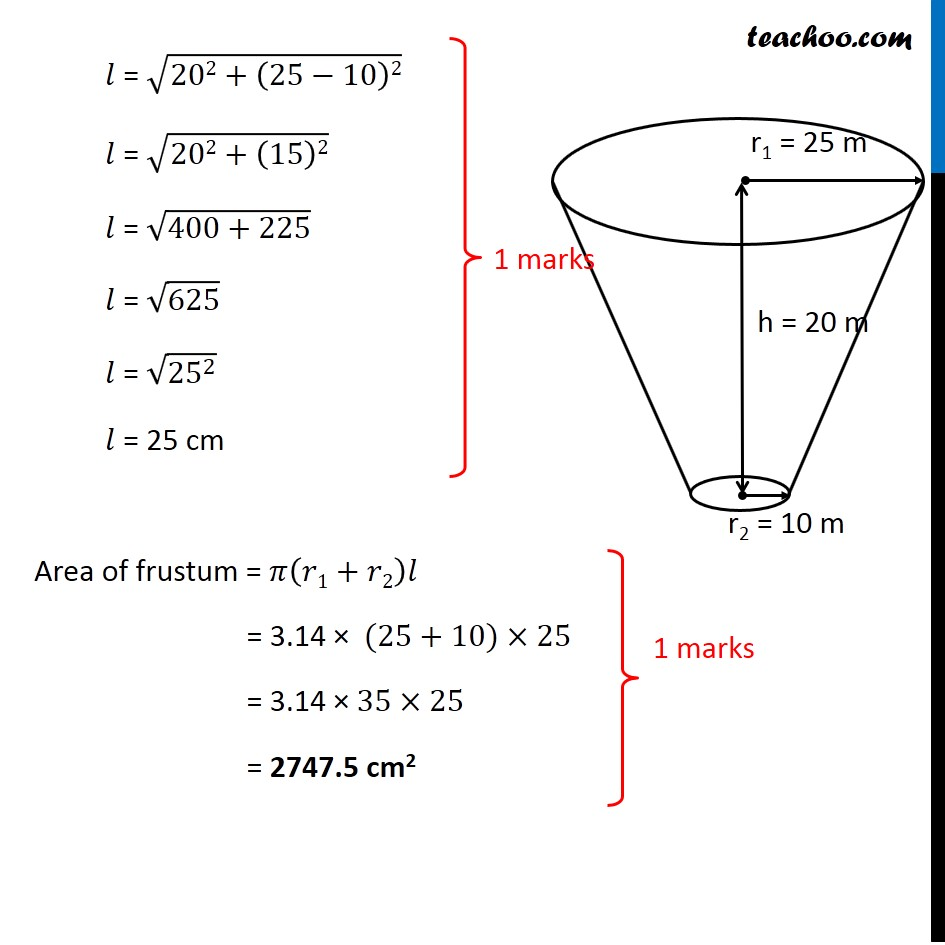CBSE Class 10 Sample Paper for 2020 Boards - Maths Standard

Class 10
Solutions of Sample Papers for Class 10 Boards

## A petrol tank is in the form of a frustum of a cone of height 20 m with diameters of its lower and upper ends as 20 m and 50 m respectively. Find the cost of petrol which can fill the tank completely at the rate of Rs. 70 per litre. Also find the surface area of the tank.Note : This is similar to Ex 13.4, 4 of NCERT – Chapter 13 Class 10

Learn in your speed, with individual attention - Teachoo Maths 1-on-1 Class

### Transcript

Question 38 (OR 1st question) A petrol tank is in the form of a frustum of a cone of height 20 m with diameters of its lower and upper ends as 20 m and 50 m respectively. Find the cost of petrol which can fill the tank completely at the rate of Rs. 70 per litre. Also find the surface area of the tank. In order to find Cost of petrol, we need to find volume (in litres) Volume of container = Volume of frustum = 1/3 𝜋ℎ(𝑟12+𝑟22+𝑟1𝑟2) Here h = height = 20 m r1 = radius of upper end = 25 m r2 = radius of lower end = 10 m Volume of container = 1/3 𝜋ℎ(𝑟12+𝑟22+𝑟1𝑟2) = 1/3×3.14×20×(252+〖10〗^2+25×10) = (3.14 × 20)/3 (625 + 100 + 250) = (3.14 × 20)/3 × 975 = 3.14 × 20 × 325 = 20410 m3 = 20410 × 1000 litre = 2,04,10,000 litre Now , Cost of 1 litre petrol = Rs 70 Cost of 2,04,10,000 litre petrol = Rs 70 × 20410000 1 m3 = 1000 litre = Rs 1428700000 Now, We need to find surface area of tank Surface Area of tank = Area of frustum = 𝜋(𝑟1+𝑟2)𝑙 Here, r1 = 20 cm , r2 = 8 cm We need to find l We know that 𝑙 = √(ℎ2+(𝑟1−𝑟2)2) 𝑙 = √(202+(25−10)2) 𝑙 = √(202+(15)2) 𝑙 = √(400+225) 𝑙 = √625 𝑙 = √(〖25〗^2 ) 𝑙 = 25 cm Area of frustum = 𝜋(𝑟1+𝑟2)𝑙 = 3.14 × (25+10)×25 = 3.14 × 35×25 = 2747.5 cm2